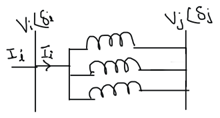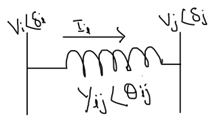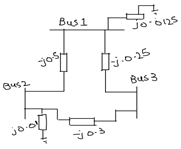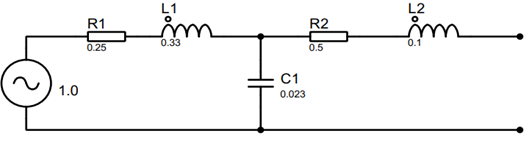check

# MCQ's Power System Analysis Fundamentals Assessment

This Power System Course aims to teach you the fundamentals of power system analysis, it makes the tedious and complex calculations done in power system studies easy for you to understand. If you think you have a good understanding of Power System Analysis, challenge yourself and take our quiz.

Question 1 of 51

A

Q/S

B

P/S

C

P/Q

Question 2 of 51

A

Watts

B

Voltage-Amperes

C

None

Question 3 of 51

A

1

B

0.707

C

0

Question 4 of 51

A

B

C

Question 5 of 51

A

0

B

0.5

C

1

Question 6 of 51

# Q.6 Why is the P.F = 1 for a purely resistive load?

A

Only reactive power is consumed

B

Only active power is consumed

C

Both a & b

Question 7 of 51

A

Q = VIcosθ

B

Q = VIsinθ

C

Q = VI

Question 8 of 51

A

B

60°

C

90°

Question 9 of 51

# Q.9 An electric current of 5A is passing through an inductive load that has a potential difference of 4KV and a lagging power factor of 0.5. What will be its apparent power at load?

A

25 KVA

B

20 KVA

C

10 KVA

Question 10 of 51

# Q.10 When we have a load of leading Power Factor, then what will be the sign of reactive power?

A

Positive

B

Negative

Question 11 of 51

# Q.11 If we increase reactive power by increasing inductive load, then what effect will occur in apparent power, current, angle, and power factor due to an increase in reactive power? (θ = Angle, I = Current, S = Apparent Power, ↑ = Increase, ↓ = Decrease).Hint: draw the power triangle and then compare

A

S ↑, θ ↑, I ↑, P.F ↑

B

S ↑, θ ↑, I ↑, P.F ↓

C

S ↑, θ ↓, I ↑, P.F ↑

Question 12 of 51

# Q.12 Why is it important to have a Power Factor close to unity? Hint: Unity Power Factor = 1.

A

A unity power factor will increase system losses and increases the current which reduces the power demand.

B

A Unity Power factor will decrease system losses and decrease the current which reduces the power demand.

C

Low P.F decrease copper loses

Question 13 of 51

# Q.13 How Capacitor will improve the power factor in inductive loads?

A

By connecting capacitor in parallel which reduces reactive power

B

By connecting capacitor in series which reduces reactive power

C

Both a & b

Question 14 of 51

# Q.14 How do synchronous motors improve the power factor?

A

At full load, the motor starts to provide reactive power because of the increase in magnetizing current which acts as an inductor.

B

At No Load, the motor starts to provide reactive power because of the increase in magnetizing current which acts as a capacitor.

C

None of these

Question 15 of 51

# Q.15 What is the formula for finding capacitance in a circuit?

A

1 / (2πfXc )

B

2πfXc

C

1/2πfC

Question 16 of 51

# Q.16 How much Power Factor is recommended for maximum efficiency and minimum power losses?

A

≤ 0.5

B

≥ 0.8

C

> 1.2

Question 17 of 51

# Q.17 If Qc > QL then what will be the sign of the angle between Real and apparent power? (Qc = Reactive Power by capacitor, QL = Reactive Power by Inductor)

A

Negative

B

Positive

Question 18 of 51

# Q.18 When Qc > QL, is the current lagging or is the voltage lagging? (Qc = Reactive Power by capacitor, QL = Reactive Power by Inductor)

A

Current Lagging

B

Voltage Lagging

Question 19 of 51

A

It is equal to the Impedance.

B

It is the square root of Impedance

C

It is the inverse of Impedance

Question 20 of 51

# Q.20 Which equation represents the admittance?

A

Y=R+jX

B

Y = G+jB

C

Y = G+jX

Question 21 of 51

# Q.21 What is the inverse of resistance?

A

Suspectance

B

Reactance

C

Conductance

Question 22 of 51

# Q.22 If we have the voltage at bus 1 is V₁ and voltage at bus 2 is V₂ and admittance between both buses is Y. What is the formula for finding current flowing between both buses?

A

I = V₁₂ * Y

B

I = V₁₂ / Y

C

I = Y / V₁₂

Question 23 of 51

# Q.23 If the voltage at bus 1 is Vᵢ and then we have multiple paths and current will flow from all paths. What will be the formula for finding total current which is also at bus 1? (n=total no of paths)A

Iᵢ = ∑ⁿⱼ₌₁ Yᵢⱼ / Vⱼ

B

Iᵢ = ∑ⁿⱼ₌₁ Yᵢⱼ * Vⱼ

Question 24 of 51

# Q.24 The voltage at bus 1 is Vᵢ ∠ δᵢ and voltage at bus 2 is Vⱼ ∠ δⱼ, If the total current passing from bus 1 is Iᵢ which is the summation of all current passing through bus 1. What will be the total apparent power at bus 1?( Iᵢ = ∑ⁿⱼ₌₁ Yᵢⱼ * Vⱼ ∠ θᵢⱼ + δⱼ )A

Sᵢ = ∑ⁿⱼ₌₁ Vᵢ * Yᵢⱼ * Vⱼ ∠δⱼ − θᵢⱼ − δᵢ

B

Sᵢ = ∑ⁿⱼ₌₁ Vᵢ * Yᵢⱼ * Vⱼ ∠δᵢ + θᵢⱼ + δⱼ

C

Sᵢ = ∑ⁿⱼ₌₁ Vᵢ * Yᵢⱼ * Vⱼ ∠δᵢ − θᵢⱼ − δⱼ

Question 25 of 51

# Q.25 If the real power entering at any bus is PG and power leaving from that bus is PL. How we can find the net real power at that bus?PB =Net real power at that Bus

A

Pʙ = Pɢ = Pʟ

B

Pʙ = Pɢ - Pʟ

C

Pʙ = Pɢ + Pʟ

Question 26 of 51

# Q.26 Why do we use the newton Raphson method in power systems?

A

For finding the impedance in a power system

B

For solving power flow equations

C

None of the above

Question 27 of 51

# Q.27 Shunt admittance in transmission lines is also referred to as..?

A

Line Charging Inductance

B

Line Charging Conductance

C

Line Charging Capacitance

Question 28 of 51

# Q.28 If impedance between two buses is j0.05 Ω. What will be admittance between these two buses?

A

-j20 Ω

B

j20 S

C

-j20 S

Question 29 of 51

# Q.29 In the Y Bus matrix, what is meant by Y₂₃?

A

Impedance between Bus 2 and Bus 3

B

Admittance between Bus 3 and Bus 2

C

Admittance between Bus 2 and Bus 3

Question 30 of 51

# Q.30 For a Y Bus Matrix, What will be the value of Y₂₃? (Given values are admittances)A

-j0.25

B

j0.3

C

-j0.3

Question 31 of 51

# Q.31 What admittance will we take for diagonal admittance in the Y Bus matrix?

A

Diagonal admittance is equal to 1 for all buses

B

Diagonal admittance is the sum of all admittances connected to that bus

C

Diagonal admittance is the sum of all admittances connected through all buses

Question 32 of 51

# Q.32 For a Y Bus Matrix, What will be the value of Y₁₁? (Given values are admittances)A

-j0.7375

B

j0.0125

C

j0.7375

Question 33 of 51

# Q.33 Which statement is correct? (Vₐ꜀ₜᵤₐₗ = Actual Voltage, Vᴘ.ᴜ = Per Unit Voltage)

A

Vₐ꜀ₜᵤₐₗ ≪ Vᴘ.ᴜ

B

Vₐ꜀ₜᵤₐₗ = Vᴘ.ᴜ

C

Vₐ꜀ₜᵤₐₗ ≫ Vᴘ.ᴜ

Question 34 of 51

# Q.34 For the circuit given below, the values given belong to which convention?A

Actual System

B

Per Unit System

Question 35 of 51

# Q.35 The actual value of impedance is 500 Ω and its base value is 1 KΩ. What will be its Per Unit impedance value?

A

2

B

0.5

C

1

Question 36 of 51

# Q.36 We have a power system having base values of 100V and 4KVA. What will be its base impedance?

A

30 Ω

B

2.5 Ω

C

25 Ω

Question 37 of 51

# Q.37 We have a step-down transformer of turns ratio 20 : 1. The base voltage on the primary side of the transformer is 1000V. What will be the base voltage on the secondary side of the transformer?

A

1000V

B

50V

C

20000V

Question 38 of 51

# Q.38 We have some base power on the primary side of the transformer. We have a step-up transformer of turns ratio 2 : 1. What will be its base power on the secondary side of the transformer?

A

Remains the same

B

Becomes Double

C

Becomes half

Question 39 of 51

# Q.39 By finding per-unit values of each component in a circuit which part of the circuit is eliminated?

A

Voltage source

B

Voltage Levels

C

Question 40 of 51

# Q.40 Which of these is not the base quantity in the per unit systems?

A

V

B

Q

C

S

D

Z

Question 41 of 51

# Q.41 In a three-phase system in Y-connection if we convert VΦ (p.u) → Vʟʟ(p.u). Then, Vʟʟ(p.u) is equals to: ( Vʟʟ(p.u) = per unit line to line voltage, VΦ (p.u) = per unit phase voltage )

A

√3 times V⌀₍ₚ.ᵤ₎

B

3 times V⌀₍ₚ.ᵤ₎

C

V⌀₍ₚ.ᵤ₎

Question 42 of 51

# Q.42 What is the formula for finding 𝐼base in a three-phase system?

A

𝐼ʙₐₛₑ = Sʙₐₛₑ / 3 * Vʙₐₛₑ

B

𝐼ʙₐₛₑ = Sʙₐₛₑ / √3 * Vʙₐₛₑ

C

𝐼ʙₐₛₑ = Sʙₐₛₑ / Vʙₐₛₑ

Question 43 of 51

# Q.43 What is the benefit of converting values into per-unit system?

A

We can reduce lengthy calculations

B

Voltage levels eliminated

C

Both a and b

Question 44 of 51

# Q.44 What benefit do we get from using per unit impedances when there is a transformer in a circuit. (N= Transformer turns ratio)

A

Per-unit Impedance at the secondary side of the transformer is N² times the per-unit impedance at the primary side of transformers.

B

Per-unit Impedance at the Primary side of the transformer is N² times the per-unit impedance at the secondary side of transformers.

C

Per-unit Impedance at the Primary side of the transformer is the same as the per-unit impedance at the secondary side of transformers

Question 45 of 51

# Q.45 If we have Zpri (p.u) = 0.8, Zpri (actual) = 8Ω,  Zpri(base) = 10Ω and transformer turns ratio is 10 : 5. What will be the value of Zsec (p.u)?

A

1.6

B

0.8

C

0.4

Question 46 of 51

# Q.46 For a system having multiple base values, how will we convert the per-unit impedance from one base to another base? (where Zp.u(2) = per-unit Impedance of new base, Zp.u(1) = per-unit Impedance according to 1ˢᵗ base)

A

Zₚ.ᵤ₍₂₎ = Zₚ.ᵤ₍₁₎ x (Vʙₐₛₑ₍₂₎² / Vʙₐₛₑ₍₁₎²) x (Sₚ.ᵤ₍₁₎ / Sₚ.ᵤ₍₂₎)

B

Zₚ.ᵤ₍₂₎ = Zₚ.ᵤ₍₁₎

C

Zₚ.ᵤ₍₂₎ = Zₚ.ᵤ₍₁₎ x (Vʙₐₛₑ₍₁₎² / Vʙₐₛₑ₍₂₎²) x (Sʙₐₛₑ₍₂₎ / Sʙₐₛₑ₍₁₎)

Question 47 of 51

# Q.47 For a balanced three-phase system, what is the hierarchy of the magnitude of the phases?

A

Phase A > Phase B > Phase C

B

Phase A = Phase B = Phase C

C

Phase A < Phase B < Phase C

Question 48 of 51

# Q.48 Which statement is correct?

A

In a balanced three-phase system, all three phases must have a difference of 60°

B

In a unbalanced three-phase system, all three phases must have a difference of 120°

C

In a balanced three-phase system, all three phases must have a difference of 120°

Question 49 of 51

# Q.49 What is the direction of rotation of all three phases in a balanced three-phase system?

A

Clockwise (CW) direction

B

Counterclockwise (CCW) direction

Question 50 of 51

# Q.50 For a balanced power system, if we have an ACB phase sequence and voltage at phase A is 480∠ 30°, what will be the voltage at Phase B? Hint: drawing the phasors will help.

A

480 ∠ 150°

B

480 ∠ 270°

C

120 ∠ 150°

Question 51 of 51

A

240 ∠ 130°

B

240 ∠ 250°

C

480 ∠ 130°